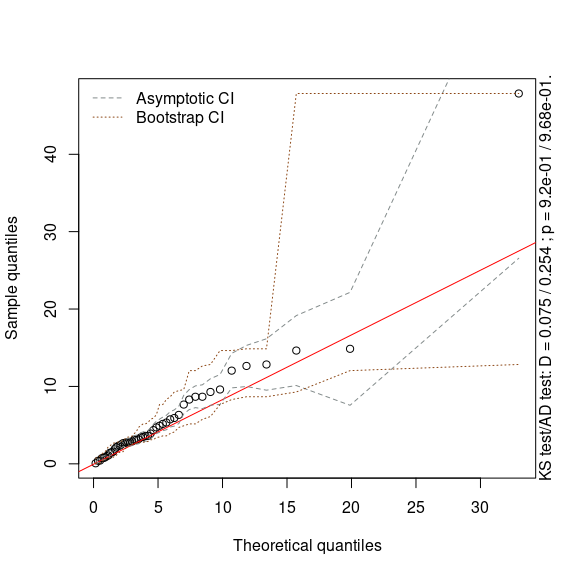# Multivariate Normal Variance Mixtures

#### 2022-01-23

library(nvmix)
library(RColorBrewer)
library(lattice)
doPDF <- FALSE
eval <- TRUE

## 1 Introduction

The R package nvmix provides functionality for (multivariate) normal variance mixture distributions, including normal and Student’s t distributions; see also Hintz et al. (2019, “Normal variance mixtures: Distribution, density and parameter estimation”). A random vector $$\mathbf{X}=(X_1,\dots,X_d)$$ follows a normal variance mixture, in notation $$\mathbf{X}\sim \operatorname{NVM}_d(\mathbf{\mu},\Sigma,F_W)$$, if, in distribution, $\mathbf{X}=\mathbf{\mu}+\sqrt{W}A\mathbf{Z},$ where $$\mathbf{\mu}\in\mathbb{R}^d$$ denotes the location (vector), $$\Sigma=AA^\top$$ for $$A\in\mathbb{R}^{d\times k}$$ denotes the scale (matrix) (a covariance matrix), and the mixture variable $$W\sim F_W$$ is a non-negative random variable independent of $$\mathbf{Z}\sim \operatorname{N}_k(\mathbf{0},I_k)$$ (where $$I_k\in\mathbb{R}^{k\times k}$$ denotes the identity matrix). Note that both the Student’s $$t$$ distribution with degrees of freedom parameter $$\nu>0$$ and the normal distribution are normal variance mixtures; in the former case, $$W\sim \operatorname{IG}(\nu/2, \nu/2)$$ (inverse gamma) and in the latter case $$W$$ is almost surely constant (taken as $$1$$ so that $$\Sigma$$ is the covariance matrix of $$\mathbf{X}$$ in this case).

Note that the density of $$\mathbf{X}$$ exists if and only if $$\Sigma$$ is positive definite and $$\mathbb{P}(W=0)=0$$. In this case one can take $$A\in\mathbb{R}^{d\times d}$$ to be the (lower triangular) Cholesky factor $$A$$ of $$\Sigma$$ such that $$AA^\top=\Sigma$$. This corresponds to the argument factor in those functions. In rnvmix(), factor is of the general form as $$A$$ above. The function pnvmix() accepts a singular scale matrix $$\Sigma$$ as input and then estimates the distribution function correctly (that is, the distribution function of the underlying singular normal variance mixture).

For most functions in the package, the quantile function of $$W$$ needs to be provided which is (here) defined as $F_W^\leftarrow(u)=\inf\{w\in[0,\infty):F_W(w)\ge u\},\quad u\in[0,1].$

## 2 Evaluating the distribution function

An important but difficult task is to evaluate the (cumulative) distribution function of a normal variance mixture distribution, so $F(\mathbf{x})=\mathbb{P}(\mathbf{X}\le\mathbf{x})=\mathbb{P}(X_1\le x_1,\dots,X_d\le x_d),\quad \mathbf{x}\in\mathbb{R}^d.$ In fact, the function pnvmix() can be used to estimate more general probabilities of the form $F(\mathbf{a},\mathbf{b} )=\mathbb{P}(\mathbf{a} < \mathbf{X}\le\mathbf{b})=\mathbb{P}(a_1 < X_1\le b_1,\dots, a_d < X_d\le b_d),\quad \mathbf{a},\mathbf{b}\in\mathbb{R}^d,$ where $$\mathbf{a}<\mathbf{b}$$ (interpreted componentwise) and entries of $$\mathbf{a},\mathbf{b}$$ are allowed to be $$\pm\infty$$. To this end, the function pnvmix() internally approximates the $$d$$-dimensional integral using a randomized Quasi Monte Carlo (RQMC) method. Due to the random nature, the result depends (slightly) on the seed .Random.seed.

### 2.1 Exponential mixture distribution

As a first example, consider a normal variance mixture with exponential mixture variable $$W$$. We illustrate two approaches how to use pnvmix() to approximate $$P(\mathbf{a} < \mathbf{X} \le \mathbf{b})$$ for randomly chosen $$\mathbf{a}\le\mathbf{b}$$.

## Generate a random correlation matrix and random limits in dimension d = 5
d <- 5
set.seed(42)
A <- matrix(runif(d * d), ncol = d)
P <- cov2cor(A %*% t(A)) # (randomly generated) correlation matrix
b <-  3 * runif(d) * sqrt(d) # (randomly generated) upper limit
a <- -3 * runif(d) * sqrt(d) # (randomly generated) lower limit

## Specify the mixture distribution parameter
rate <- 1.9 # exponential rate parameter

## Method 1: Use R's qexp() function and provide a list as 'mix'
set.seed(42)
(p1 <- pnvmix(b, lower = a, qmix = list("exp", rate = rate), scale = P))
##  0.5213731
## attr(,"abs. error")
##  0.0004675596
## attr(,"rel. error")
##  0.0008967852
## attr(,"numiter")
##  2
## Method 2: Define the quantile function manually (note that
##           we do not specify rate in the quantile function here,
##           but conveniently pass it via the ellipsis argument)
set.seed(42)
(p2 <- pnvmix(b, lower = a, qmix = function(u, lambda) -log(1-u)/lambda,
scale = P, lambda = rate))
##  0.5213731
## attr(,"abs. error")
##  0.0004675596
## attr(,"rel. error")
##  0.0008967852
## attr(,"numiter")
##  2
## Comparison
stopifnot(all.equal(p1, p2))

We see that the results coincide.

If higher precision of the computed probabilities is desired, this can be accomplished by changing the argument pnvmix.abstol (which defaults to 1e-3) in the control argument at the expense of a higher run time.

pnvmix(b, lower = a, qmix = function(u, lambda) -log(1-u)/lambda,
lambda = rate, scale = P, control = list(pnvmix.abstol = 1e-5))
##  0.5211905
## attr(,"abs. error")
##  8.854015e-06
## attr(,"rel. error")
##  1.698806e-05
## attr(,"numiter")
##  160

### 2.2 Three-point mixture distribution

As a next example, consider a normal variance mixture where $$W$$ is discrete. This time, we are interested in computing the one-sided probabability $$\mathbb{P}(\mathbf{X}\le\mathbf{b})=F(\mathbf{b})$$ for $$\mathbf{b}$$ as constructed before.

## Define the quantile function of the three-point distribution
## which puts masses 'p' at the numbers 'x'
x <- c(1, 3, 5) # support
p <- c(0.2, 0.3, 0.5) # probabilities
qW <- function(u)
(u <= p) * x + (u > p & u <= p+p) * x + (u > p+p) * x

## Call pnvmix(); lower defaults to (-Inf,...,-Inf)
set.seed(42)
(p1 <- pnvmix(b, qmix = qW, scale = P))
##  0.8968747
## attr(,"abs. error")
##  0.0003311867
## attr(,"rel. error")
##  0.0003692676
## attr(,"numiter")
##  1

This could have also been obtained as follows but we would have called pNorm() (so pnvmix()) three times then.

set.seed(42)
p2 <- sum(sapply(1:3, function(k) p[k] * pNorm(b, scale = x[k] * P)))
stopifnot(all.equal(p1, p2, check.attributes = FALSE, tol = 5e-4))

### 2.3 The wrappers pNorm() and pStudent()

For the two special cases of Student’s $$t$$ distribution and the normal distribution, pNorm() and pStudent() are user-friendly wrappers of pnvmix(). Note that pStudent() works for any degree of freedom parameter $$\nu>0$$ (not necessarily integer) – to the best of our knowledge, this functionality was not available in R at the time of development of this package.

### 2.4 The effect of algorithm-specific parameters

The function pnvmix() (and thus the wrappers pStudent() and pNorm()) give the user the possibility to change algorithm-specific parameters via the control argument. A few of them are (for others see ?get_set_param):

• method: The integration method to be used. The default, a randomized Sobol sequence, has proven to outperform the others.
• precond: A logical variable indicating whether a preconditioning step, that is, a reordering of the integration limits (and related rows and columns of scale) is to be performed. If TRUE, the reordering is done in a way such that the expected lengths of the integration limits is increasing going from the outermost to the innermost integral. In the vast majority of cases, this leads to a decrease in the variance of the integrand and thus to a decrease in computational time.
• mean.sqrt.mix: $$E(\sqrt{W})$$. This number is needed for the preconditioning. In case of Student’s $$t$$ and the normal distribution, this value is calculated internally. For all other cases this value is estimated internally if not provided.
• increment: Determines how large the next point set should be if the previous point set was not large enough to ensure the specified accuracy. When "doubling" is used, there will be as many additional points as there were in the previous iteration and if "num.init" is used, there will be fun.eval additional points in each iteration. The former option (default) will lead to slightly more accurate results at the cost of slightly higher run time.

Let us now illustrate the effect of method and precond on the performance of pnvmix() with mix = 'inverse.gamma'. To this end we use the wrapper pStudent(). We set pnvmix.abstol = NULL so that the algorithm runs until the number of function evaluations exceeds fun.eval. We do this for different values of fun.eval in order to get an idea of the speed of convergence. We also compute the regression coefficients which act as a summary measure of the speed of convergence.

## Setup
df <- 1.5 # degrees of freedom
maxiter <- 9 # note: i iterations require 3 * 2^8 * 2^i function evaluations
max.fun.evals <- 3 * 2^8 * 2^seq(from = 2, to = maxiter, by = 1)
errors <- matrix(, ncol = length(max.fun.evals), nrow = 4)
nms <- c("Sobol  with preconditioning", "Sobol  w/o  preconditioning",
"PRNG with preconditioning", "PRNG w/o  preconditioning")
rownames(errors) <- nms

## Computing the errors
## Note:
## - resetting the seed leads to a fairer comparison here
## - set 'verbose' to 0 or FALSE to avoid warnings which inevitably occur
##   due to 'pnvmix.abstol = NULL'
for(i in seq_along(max.fun.evals)) {
N.max <- max.fun.evals[i]
## Sobol with preconditioning
set.seed(42)
errors[nms,i] <-
attr(pStudent(b, lower = a, scale = P,  df = df,
control = list(pnvmix.abstol = NULL, fun.eval = c(2^6, N.max)),
verbose = FALSE), "abs. error")
## Sobol without preconditioning
set.seed(42)
errors[nms,i] <-
attr(pStudent(b, lower = a, scale = P,  df = df,
control = list(precond = FALSE, pnvmix.abstol = NULL,
fun.eval = c(2^6, N.max)),
verbose = FALSE), "abs. error")
## PRNG with preconditioning
set.seed(42)
errors[nms,i] <-
attr(pStudent(b, lower = a, scale = P,  df = df,
control = list(method = "PRNG", pnvmix.abstol = NULL,
fun.eval = c(2^6, N.max)),
verbose = FALSE), "abs. error")
## PRNG without preconditioning
set.seed(42)
errors[nms,i] <-
attr(pStudent(b, lower = a, scale = P,  df = df,
control = list(method = "PRNG", precond = FALSE,
pnvmix.abstol = NULL, fun.eval = c(2^6, N.max)),
verbose = FALSE), "abs. error")
}

## Computing the regression coefficients
coeff <- apply(errors, 1, function(y) lm(log(y) ~ log(max.fun.evals))$coeff) names(coeff) <- nms ## Plot if(doPDF) pdf(file = (file <- "fig_pnvmix_error_comparison.pdf"), width = 7, height = 7) pal <- colorRampPalette(c("#000000", brewer.pal(8, name = "Dark2")[c(7, 3, 5)])) cols <- pal(4) # colors plot(NA, log = "xy", xlim = range(max.fun.evals), ylim = range(errors), xlab = "Number of function evaluations", ylab = "Estimated error") lgnd <- character(4) for(k in 1:4) { lines(max.fun.evals, errors[k,], col = cols[k]) lgnd[k] <- paste0(nms[k]," (",round(coeff[k], 2),")") } legend("topright", bty = "n", lty = rep(1, 4), col = rev(cols), legend = rev(lgnd)) if(doPDF) dev.off()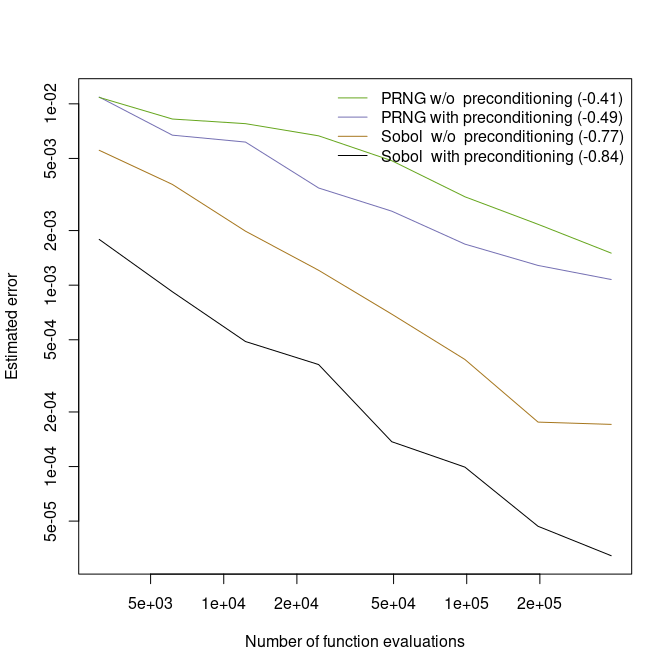We can see that in this example Sobol’ outperforms PRNG and that the preconditioning helps significantly in reducing the error. ## 3 Evaluating the density function Another important task is to evaluate the density function of a normal variance mixture. This is particularly important for likelihood-based methods. In general, the density is given in terms of a univariate integral which the function dnvmix() internally approximates using a randomized Quasi Monte Carlo (RQMC) method. Due to the random nature, the result slightly varies with .Random.seed. Note that if $$\Sigma$$ is singular, the density does not exist. Note the argument log in dnvmix(): Rather than estimating the density, the logarithmic density is estimated. Only if log = FALSE (the default), the actual density is returned. This is usually numerically more stable than estimating the density and then applying the logarithm to the computed density. Also note that for many applications, the log-density is the actual quantity of interest, for example, when computing the log-likelihood. We give two small examples: ### 3.1 3-point mixture x <- matrix(1:15/15, ncol = d) # evaluation points of the density set.seed(1) (d1 <- dnvmix(x, qmix = qW, scale = P)) # computed density values ##  7.301123e-15 6.122256e-15 4.975161e-15 ## attr(,"abs. error") ##  1.440302e-24 1.207745e-24 9.814566e-25 ## attr(,"rel. error") ##  1.972713e-10 1.972713e-10 1.972713e-10 ## attr(,"numiter") ##  1 1 1 set.seed(1) (d2 <- dnvmix(x, qmix = qW, scale = P, log = TRUE)) # log-density ##  -32.55075 -32.72685 -32.93432 ## attr(,"abs. error") ##  1.972712e-10 1.806429e-10 1.628447e-10 ## attr(,"rel. error") ##  6.060421e-12 5.519716e-12 4.944528e-12 ## attr(,"numiter") ##  1 1 1 stopifnot(all.equal(d1, exp(d2), check.attributes = FALSE)) # check ## This could have also been obtained via d3 <- rowSums(sapply(1:3, function(k) p[k] * dNorm(x, scale = x[k] * P))) stopifnot(all.equal(d1, d3, tol = 1e-10, check.attributes = FALSE)) ### 3.2 Inverse-gamma mixture In the case of an inverse-gamma mixture (so that $$\mathbf{X}$$ is multivariate $$t$$), the density is known. This can be used to accurately estimate the error in our estimation procedure, as illustrated here: n <- 40 # sample size x <- matrix(1:n, ncol = 2) # n/2 - two dimensional evaluation points m <- mahalanobis(x, center = c(0,0), cov = diag(2)) # for plotting df <- 2 d3.1 <- dStudent(x, df = df, log = TRUE) # true value ## Specify 'qmix' as function to force estimation of log-density via RQMC d3.2 <- dnvmix(x, qmix = function(u) 1/qgamma(1-u, shape = df/2, rate = df/2), log = TRUE) rel.err <- (d3.2 - d3.1) / d3.1 stopifnot(max(abs(rel.err)) < 5e-3) # check cols <- pal(2) if(doPDF) pdf(file = (file <- paste0("fig_dStudentvsdnvmix.pdf")), width = 6, height = 6) plot(sqrt(m), d3.1, type = 'l', col = cols, xlab = expression(paste("Mahalanobis Distance ", x^T, x)), ylab = "log-density") lines(sqrt(m), d3.2, col = cols, lty = 2) legend("topright", c("True log-density", "Estimated log-density"), lty = c(1,2), col = cols[1:2], bty = 'n') if(doPDF) dev.off()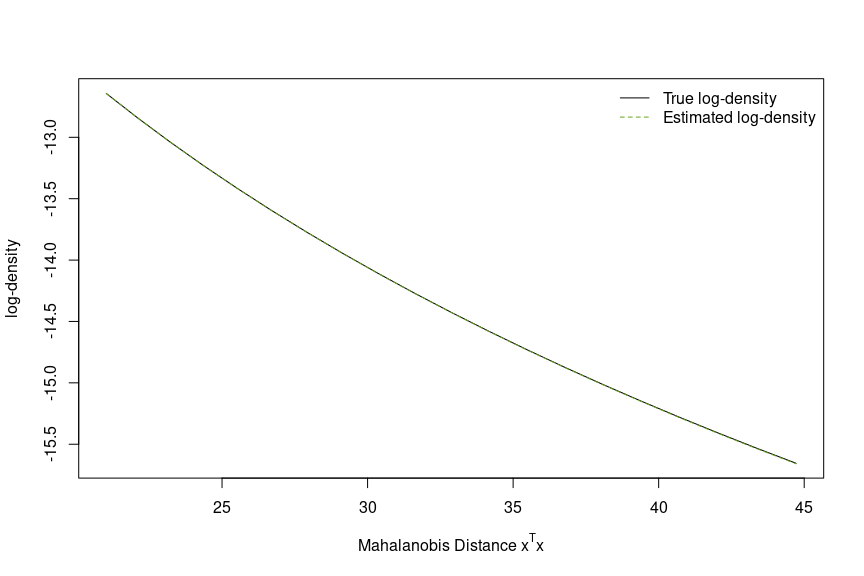## 4 (Quasi-)random number generation The function rnvmix() provides a flexible tool to sample from (multivariate) normal variance mixtures. The structure is similar to the one of dnvmix() and pnvmix() (but also different in some aspects; see ?dnvmix). The user can specify the argument qmix which, as usual, corresponds to the quantile function $$F_W^\leftarrow$$ of $$W$$ or, alternatively, the argument rmix, which corresponds to a random number generator for $$W$$. This is due to the fact that there are distributions for which it is hard to find the quantile function, but for which sampling procedures exist (for example, for stable distributions). As an example call of rnvmix(), let us revisit Section 2.1 where $$W\sim\mathrm{Exp}(1.9)$$. ## Sampling n <- 500 # sample size set.seed(42) r1 <- rnvmix(n, rmix = list("exp", rate = rate)) # uses the default P = diag(2) ## Plot if(doPDF) pdf(file = (file <- paste0("fig_rnvmix_W_exp.pdf")), width = 6, height = 6) plot(r1, xlab = expression(X), ylab = expression(X)) if(doPDF) dev.off()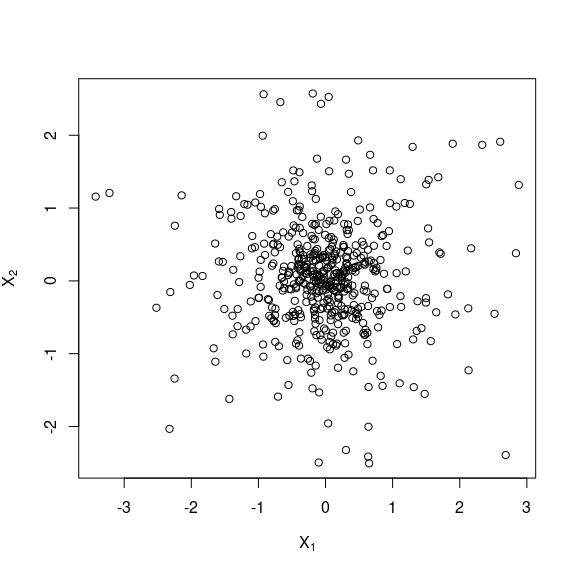An important argument of rnvmix() is method. This can be either "PRNG" (classical pseudo-random sampling) or "sobol" or "ghalton" (for the inversion method based on the corresponding low-discrepancy point set). If method is not "PRNG", qmix must be provided. As an example, let us revisit Section 2.2 where $$W$$ was following a three-point distribution. ## Sampling set.seed(42) r1 <- rnvmix(n, qmix = qW) r2 <- rnvmix(n, qmix = qW, method = "ghalton") ## Plot if(doPDF) pdf(file = (file <- paste0("fig_rnvmix_W_three-point.pdf")), width = 9, height = 6) ran <- range(r1, r2) opar <- par(pty = "s") layout(t(1:2)) plot(r1, xlab = expression(X), ylab = expression(X), main = "Pseudo-random sample", xlim = ran, ylim = ran) plot(r2, xlab = expression(X), ylab = expression(X), main = "Quasi-random sample", xlim = ran, ylim = ran) layout(1) par(opar) if(doPDF) dev.off()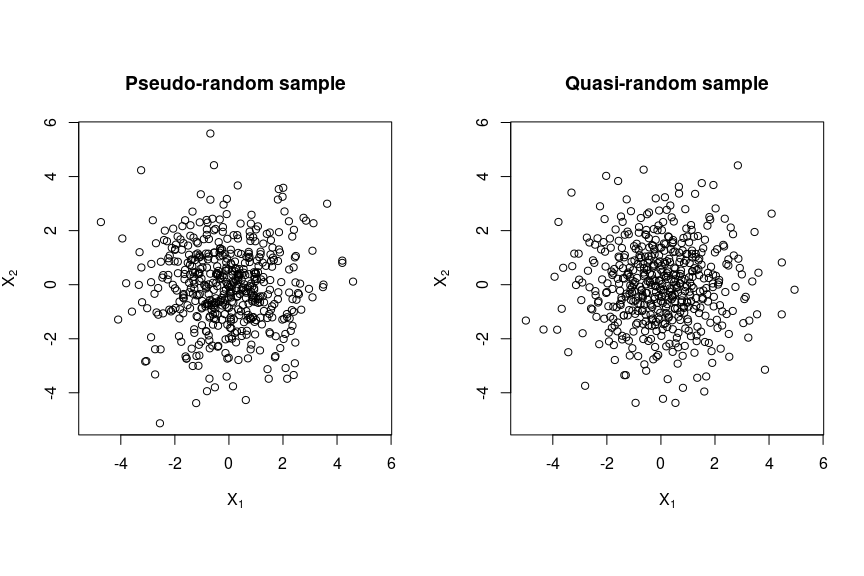When $$W$$ is discrete and has finite support, one can also easily sample from the corresponding normal variance mixture using rNorm(). ## Sampling set.seed(42) r <- lapply(1:3, function(k) rNorm(p[k] * n, scale = diag(x[k], 2))) ## Plot if(doPDF) pdf(file = (file <- paste0("fig_rnvmix_W_three-point_via_rNorm.pdf")), width = 6, height = 6) ran <- range(r) cols <- pal(4) opar <- par(pty = "s") plot(NA, xlim = ran, ylim = ran, xlab = expression(X), ylab = expression(X)) for(k in 1:3) points(r[[k]], col = cols[k+1]) par(opar) if(doPDF) dev.off()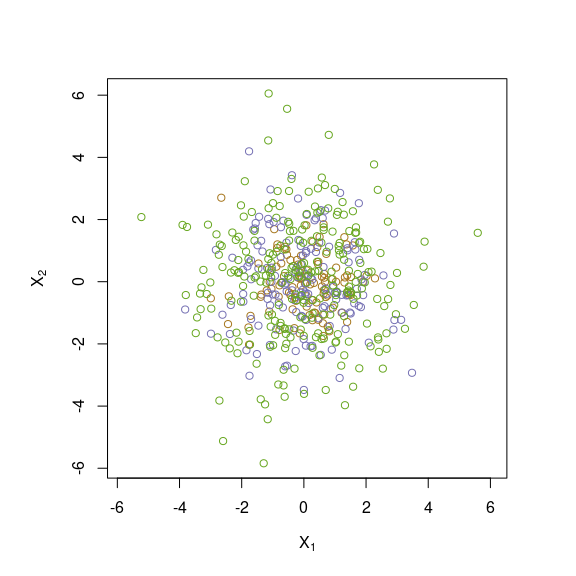This examples helps understanding normal variance mixtures. Note that the brown points come from $$\operatorname{N}(\mathbf{0}, I_2)$$, the blue ones from $$\operatorname{N}(\mathbf{0}, 3I_2)$$ and the green ones from $$\operatorname{N}(\mathbf{0}, 5I_2)$$ and that their frequencies correspond to the probabilities $$\mathbb{P}(W=1)$$, $$\mathbb{P}(W=3)$$ and $$\mathbb{P}(W=5)$$. Unlike dnvmix(), rnvmix() can handle singular normal variance mixtures. In this case, the matrix factor (which is a matrix $$A\in\mathbb{R}^{d\times k}$$ such that $$AA^\top=\Sigma$$) has to be provided. In the following example, we consider a Student’s $$t$$ distribution via the wrapper rStudent(). As expected in the singular case, all points lie on a plane which is visible after a suitable rotation of the cloud plot. ## Sampling df <- 3.9 # degrees of freedom factor <- matrix(c(1,0, 0,1, 0,1), ncol = 2, byrow = TRUE) # (3,2)-matrix 'factor' Sigma <- tcrossprod(factor) # the 'scale' corresponding to factor stopifnot(Sigma == factor %*% t(factor)) set.seed(42) r <- rStudent(n, df = df, factor = factor) # sample ## Plot if(doPDF) pdf(file = (file <- paste0("fig_rnvmix_singular.pdf")), width = 6, height = 6) cloud(r[,3] ~ r[,1] * r[,2], screen = list(z = 115, x = -68), xlab = expression(X), ylab = expression(X), zlab = expression(X), scales = list(arrows = FALSE, col = "black"), par.settings = modifyList(standard.theme(color = FALSE), list(axis.line = list(col = "transparent"), clip = list(panel = "off")))) if(doPDF) dev.off()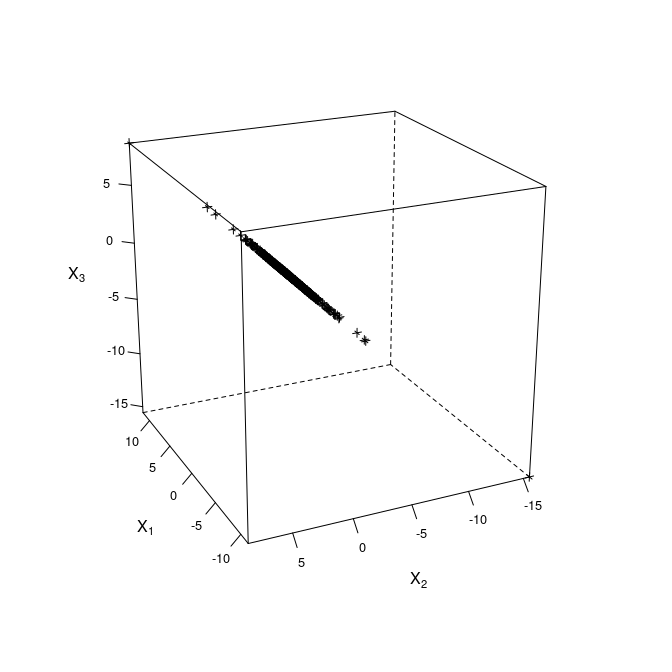## 5 Parameter estimation The function fitnvmix() can be used to fit any multivariate normal variance mixture distribution to data so long as the quantile function of the mixing variable $$W$$ is available. Internally, an ECME (Expectation/Conditional Maximization Either) algorithm is used to estimate the mixing parameters of $$W$$, the location vector $$\mathbf{\mu}$$ and the scale matrix $$\Sigma$$. The specification of $$W$$ is passed to fitnvmix() via the argument qmix, see also the documentation for further details. Here, qmix can be either a function of $$u$$ and $$\nu$$ (where $$\nu$$ corresponds to the parameters of the mixing random variable $$W$$) or a string (currently allowed are qmix = "constant", qmix = "inverse.gamma" and qmix = "pareto"); note that in the latter case, analytical formulas for densities and weights are used where as in the former case, all densities and weights are estimated via RQMC methods. The following example illustrates the problem of fitting data to a Pareto-mixture. set.seed(42) # for reproducibility ## Define 'qmix' as the quantile function of a Par(nu, 1) distribution qmix <- function(u, nu) (1-u)^(-1/nu) ## Parameters for sampling n <- 50 d <- 3 loc <- rep(0, d) # true location vector A <- matrix(runif(d * d), ncol = d) scale <- cov2cor(A %*% t(A)) # true scale matrix nu <- 2.4 # true mixing parameter mix.param.bounds <- c(1, 10) # nu in [1, 10] ## Sample data using 'rnvmix()': x <- rnvmix(n, qmix = qmix, nu = nu, loc = loc, scale = scale) ## Call 'fitvnmix()' with 'qmix' as function (so all densities/weights are estimated) (MyFit21 <- fitnvmix(x, qmix = qmix, mix.param.bounds = mix.param.bounds)) ## Call: fitnvmix(x = x, qmix = qmix, mix.param.bounds = mix.param.bounds) ## Input data: 50 3-dimensional observations. ## Normal variance mixture specified through quantile function of the mixing variable ## function (u, nu) (1 - u)^(-1/nu) ## with unknown 'loc' vector and unknown 'scale' matrix. ## Approximated log-likelihood at reported parameter estimates: -100.751700 ## Termination after 23 iterations, convergence detected. ## Estimated mixing parameter(s) 'nu': ##  2.198 ## Estimated 'loc' vector: ##  -0.1198 -0.0423 -0.1724 ## Estimated 'scale' matrix: ## [,1] [,2] [,3] ## [1,] 1.338 1.2723 1.1614 ## [2,] 1.272 1.3910 0.9409 ## [3,] 1.161 0.9409 1.1637 ## Call 'fitnvmix()' with 'qmix = "pareto"' in which case an analytical formula ## for the density is used (MyFit22 <- fitnvmix(x, qmix = "pareto", mix.param.bounds = mix.param.bounds)) ## Call: fitnvmix(x = x, qmix = "pareto", mix.param.bounds = mix.param.bounds) ## Input data: 50 3-dimensional observations. ## Normal variance mixture specified through quantile function of the mixing variable ## "pareto" ## with unknown 'loc' vector and unknown 'scale' matrix. ## log-likelihood at reported parameter estimates: -100.752900 ## Termination after 11 iterations, convergence detected. ## Estimated mixing parameter(s) 'nu': ##  2.195 ## Estimated 'loc' vector: ##  -0.11978 -0.04222 -0.17244 ## Estimated 'scale' matrix: ## [,1] [,2] [,3] ## [1,] 1.338 1.272 1.161 ## [2,] 1.272 1.391 0.941 ## [3,] 1.161 0.941 1.164 stopifnot(all.equal(MyFit21$nu, MyFit22$nu, tol = 5e-2)) ## Produce a Q-Q-Plot of the sampled mahalanobis distance versus their theoretical ## quantiles with parameters estimated in 'MyFit21' if(doPDF) pdf(file = (file <- paste0("fig_fitnvmix_qqplot.pdf")), width = 6, height = 6) qqplot_maha(x, qmix = "pareto", loc = MyFit21$loc, scale = MyFit21$scale, alpha = MyFit21$nu)
if(doPDF) dev.off()